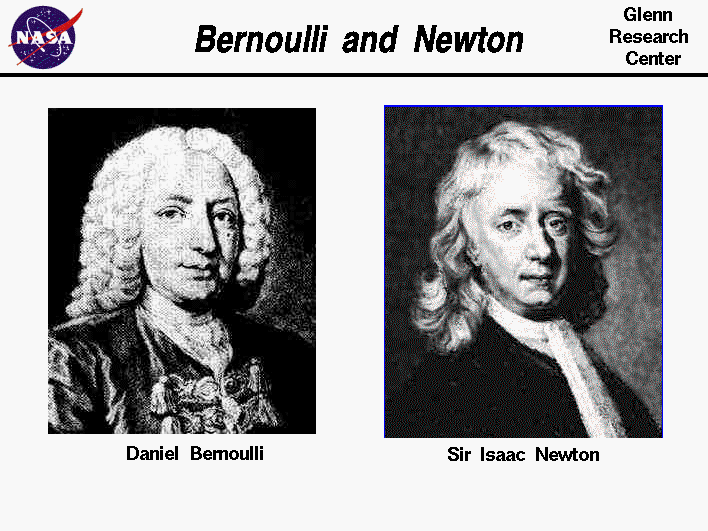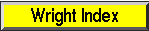+ Text Only Site
+ Non-Flash Version
+ Contact GlennLift is the force that holds an aircraft in the air. How is lift generated? There are many explanations for the generation of lift found in encyclopedias, in basic physics textbooks, and on Web sites. Unfortunately, many of the explanations are misleading and incorrect. Theories on the generation of lift have become a source of great controversy and a topic for heated arguments for many years. The proponents of the arguments usually fall into two camps: (1) those who support the "Bernoulli" position that lift is generated by a pressure difference across the wing, and (2) those who support the "Newton" position that lift is the reaction force on a body caused by deflecting a flow of gas. Notice that we place the names in quotation marks because neither Newton nor Bernoulli ever attempted to explain the aerodynamic lift of an object. The names of these scientists are just labels for two camps. Looking at the lives of Bernoulli and Newton we find more similarities than differences. On the figure at the top of this page we show portraits of Daniel Bernoulli, on the left, and Sir Isaac Newton, on the right. Newton worked in many areas of mathematics and physics. He developed the theories of gravitation in 1666, when he was only 23 years old. Some twenty years later, in 1686, he presented his three laws of motion in the Principia Mathematica Philosophiae Naturalis . He and Gottfried Leibnitz are also credited with the development of the mathematics of Calculus. Bernoulli also worked in many areas of mathematics and physics and had a degree in medicine. In 1724, at age 24, he had published a mathematical work in which he investigated a problem begun by Newton concerning the flow of water from a container and several other problems involving differential equations. In 1738, his work Hydrodynamica was published. In this work, he applied the conservation of energy to fluid mechanics problems. Which camp is correct? How is lift generated? When a gas flows over an object, or when an object moves through a gas, the molecules of the gas are free to move about the object; they are not closely bound to one another as in a solid. Because the molecules move, there is a velocity associated with the gas. Within the gas, the velocity can have very different values at different places near the object. Bernoulli's equation, which was named for Daniel Bernoulli, relates the pressure in a gas to the local velocity; so as the velocity changes around the object, the pressure changes as well. Adding up (integrating) the pressure variation times the area around the entire body determines the aerodynamic force on the body. The lift is the component of the aerodynamic force which is perpendicular to the original flow direction of the gas. The drag is the component of the aerodynamic force which is parallel to the original flow direction of the gas. Now adding up the velocity variation around the object instead of the pressure variation also determines the aerodynamic force. The integrated velocity variation around the object produces a net turning of the gas flow. From Newton's third law of motion, a turning action of the flow will result in a re-action (aerodynamic force) on the object. So both "Bernoulli" and "Newton" are correct. Integrating the effects of either the pressure or the velocity determines the aerodynamic force on an object. We can use equations developed by each of them to determine the magnitude and direction of the aerodynamic force. What is the argument? Arguments arise because people mis-apply Bernoulli and Newton's equations and because they over-simplify the description of the problem of aerodynamic lift. The most popular incorrect theory of lift arises from a mis-application of Bernoulli's equation. The theory is known as the "equal transit time" or "longer path" theory which states that wings are designed with the upper surface longer than the lower surface, to generate higher velocities on the upper surface because the molecules of gas on the upper surface have to reach the trailing edge at the same time as the molecules on the lower surface. The theory then invokes Bernoulli's equation to explain lower pressure on the upper surface and higher pressure on the lower surface resulting in a lift force. The error in this theory involves the specification of the velocity on the upper surface. In reality, the velocity on the upper surface of a lifting wing is much higher than the velocity which produces an equal transit time. If we know the correct velocity distribution, we can use Bernoulli's equation to get the pressure, then use the pressure to determine the force. But the equal transit velocity is not the correct velocity. Another incorrect theory uses a Venturi flow to try to determine the velocity. But this also gives the wrong answer since a wing section isn't really half a Venturi nozzle. There is also an incorrect theory which uses Newton's third law applied to the bottom surface of a wing. This theory equates aerodynamic lift to a stone skipping across the water. It neglects the physical reality that both the lower and upper surface of a wing contribute to the turning of a flow of gas. The real details of how an object generates lift are very complex and do not lend themselves to simplification. For a gas, we have to simultaneously conserve the mass, momentum, and energy in the flow. Newton's laws of motion are statements concerning the conservation of momentum. Bernoulli's equation is derived by considering conservation of energy. So both of these equations are satisfied in the generation of lift; both are correct. The conservation of mass introduces a lot of complexity into the analysis and understanding of aerodynamic problems. For example, from the conservation of mass, a change in the velocity of a gas in one direction results in a change in the velocity of the gas in a direction perpendicular to the original change. This is very different from the motion of solids, on which we base most of our experiences in physics. The simultaneous conservation of mass, momentum, and energy of a fluid (while neglecting the effects of air viscosity) are called the Euler Equations after Leonard Euler. Euler was a student of Johann Bernoulli, Daniel's father, and for a time had worked with Daniel Bernoulli in St. Petersburg. If we include the effects of viscosity, we have the Navier-Stokes Equations which are named after two independent researchers in France and in England. To truly understand the details of the generation of lift, one has to have a good working knowledge of the Euler Equations. Activities:Guided ToursTheories of Lift:Navigation ..Beginner's Guide Home Page+ Inspector General Hotline + Equal Employment Opportunity Data Posted Pursuant to the No Fear Act + Budgets, Strategic Plans and Accountability Reports + Freedom of Information Act + The President's Management Agenda + NASA Privacy Statement, Disclaimer, and Accessibility CertificationEditor: Nancy Hall NASA Official: Nancy Hall Last Updated: May 05 2015 + Contact Glenn